Support the Monkey! Tell All your Friends and TeachersHome MonkeyNotes Printable Notes Digital Library Study Guides Study Smart Parents Tips College Planning Test Prep Fun Zone Help / FAQ How to Cite Request a New Title

Thus in   2 ´ 2 table df = ( 2 - 1 ) ( 2 - 1 ) = 1

3 ´ 3 table df = (3 - 1 ) ( 3 - 1 ) = 4

4 ´ 4 table df = ( 4 - 1 ) ( 4 - 1 ) = 9 etc.

If the data is not in the form of contingency tables but as a series of individual observations or discrete or continuous series then it is calculated by n = n - 1 where n is the number of frequencies or values of number of independent individuals.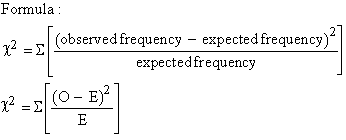where O = observed frequency and E = expected frequency.

Example The following table shows the age groups of people interviewed according to their age-group and the number in each group estimated to have T. B.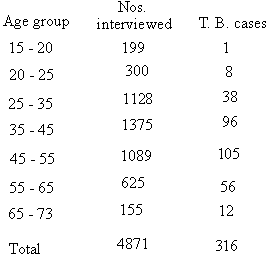Do these figures justify the hypothesis that T. B. is equally popular in all age groups ?Your browser does not support the IFRAME tag.

Solution: If T.B. equally popular in all groups then in each age group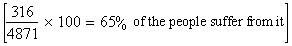.

O n this basis the observed and expected frequencies would be as

 Age group Observed Cases Expected cases 15 - 20 1 13 20 - 25 8 19.5 25 - 35 38 73 35 - 45 96 89 45 - 55 105 71 55 - 65 56 40.5 65 - 75 12 10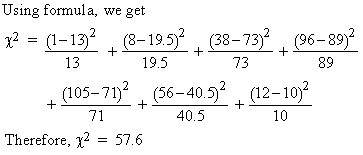Also n (df ) = ( c - 1 ) ( n - 1 ) = ( 7 - 1 ) ( 2 - 1 ) = 6

From the c2 - table, c2 0.05, n = 6 = 12.59

Since 57.6 > 12.59 at 0.05 level of significance for 6 degree of freedom, the difference is significant and the hypothesis is not justified.

Example 12 die were thrown 4096 times and a throw of 6 was reckoned as a success. The observed frequencies were as given below :
 Number of successes 0 1 2 3 4 5 6 7 and over Total Frequencies 447 1145 1181 796 380 115 24 8 - 4096

Find the value of c2 on the hypothesis that the dice were unbiased and hence show that the data is consistent with the hypothesis so far as the c2 test is concerned.

Solution: On the hypothesis of unbiased dice the theoretical frequencies in 4096 throws are the terms in the Binomial expansion of 4096 ( 5/6 + 1/6 )2 and are given below :

 Number of successes 0 1 2 3 4 5 6 7 and over Total Theoretical Frequencies 459 1102 1212 808 364 116 27 8 - 4096

Using the formula :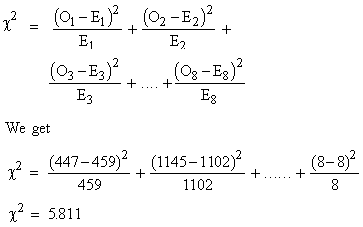The number of classes is 8 but the total of the observed and theoretical frequencies agree. Therefore n (df) = 8 - 1 = 7. From the table c20.05, n = 7 = 14.07 i.e. the calculated value of c2 is not significant and thus the observed frequency distribution is consistent with the hypothesis.

Example Two hundreds digits were chosen at random from a set of tables. The frequencies of the digits are as below :

Index

8.1 Population
8.2 Sample
8.3 Parameters and Statistic
8.4 Sampling Distribution
8.5 Sampling Error
8.6 Central Limit Theorem
8.7 Critical Region
8.8 Testing of Hypothesis
8.9 Errors in Tesitng of Hypothesis
8.10 Power o a Hypothesis Test
8.11 Sampling of Variables
8.12 Sampling of Attributes
8.13 Estimation
8.14 Testing the Difference Between Means
8.15 Test for Difference Between Proportions
8.16 Two Tailed and one Tailed Tests
8.17 Test of Significance for Small Samples
8.18 Students t-distribution
8.19 Distribution of 't' for Comparison of Two Samples Means Independent Samples
8.20 Testing Difference Between Mens of Two Samples Dependent Samples or Matched Paired Observations
8.21 Chi-Square
8.22 Sampling Theory of Correlation
8.23 Sampling Theory of Regression

Chapter 1Search: All Products Books Popular Music Classical Music Video DVD Toys & Games Electronics Software Tools & Hardware Outdoor Living Kitchen & Housewares Camera & Photo Cell Phones Keywords: